Weil algebra of a Lie algebra

Letbe a connected Lie group with Lie algebra. The Weil algebraofwas first introduced in a series of seminars by H. Cartan [a1], in part based on some unpublished work of A. Weil. As a differential graded algebra, it is given by the tensor product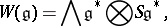where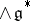and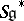denote the exterior and symmetric algebras, respectively (cf. also Exterior algebra; Symmetric algebra).

The Weil algebra and its generalizations have been studied extensively by F.W. Kamber and Ph. Tondeur [a3] [a4]. Let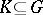be a maximal compact subgroup, withdenoting the Lie algebra of. The relative Weil algebra foris defined byWith regards to the universal classifying bundle(cf. also Bundle; Classifying space; Universal space), there are canonical isomorphisms in cohomologywhere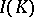denotes the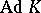-invariant polynomials. For a given integer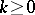, one has the ideal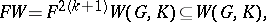generated by, for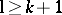. This leads to the truncated Weil algebra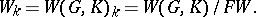The cohomology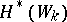plays a prominent role in the study of secondary characteristic classes (cf. also Characteristic class) of foliations and foliated bundles [a3] (see also [a2]).